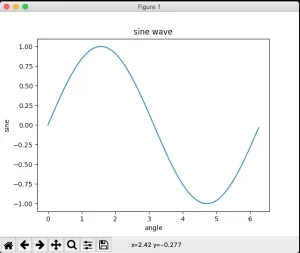# How To Draw A Simple Sine Curve Using Python Matplotlib

In this article, you will learn how to use Matplotlib to plot a simple sine curve that shows the relationship between the angle and the value of the sine function.

### 1. How To Draw A Simple Sine Curve Using Python Matplotlib Steps.

1. First, import the Pyplot module in the Matplotlib package, and simplify the name of the imported package in the form of as alias.
`import matplotlib.pyplot as plt`
2. Next, use the function arange() provided by NumPy to create a set of data to draw the image.
```# import numpy library.
import numpy as np

# get the ndarray object between 0 and 2π.
x = np.arange(0, math.pi*2, 0.05)```
3. The value of X obtained above is the value on the X-axis, and the sinusoidal value corresponding to this value, that is, the Y-axis value is obtained by the following method.
`y = np.sin(x)`
4. Use the plot() function to plot the sine curve with the x and y value.
`plt.plot(x,y)`
5. The main drawing work has been completed, but we still need to draw some details, which need to be supplemented, such as x-axis and y-axis labels, image titles, etc.
```# plot the x, y label.
plt.xlabel("angle")
plt.ylabel("sine")

# plot the image title.
plt.title('sine wave')```
6. The complete example program code is as follows.
```# import matplotlib pyplot module.
from matplotlib import pyplot as plt

# import numpy module.
import numpy as np

# import python math module.
import math

# call the math.pi method to convert radians to angles.
# get the x-axis value.
x = np.arange(0, math.pi*2, 0.05)
# calculate the y-axis value use the np.sin() method.
y = np.sin(x)

# plot the sine curve.
plt.plot(x,y)

# draw the x-axis and y-axis label.
plt.xlabel("angle")
plt.ylabel("sine")

# draw the image title.
plt.title('sine wave')

# call the show() method to display the image.
plt.show()```
7. When you run the above example source code, you will get the below sine curve image.References

This site uses Akismet to reduce spam. Learn how your comment data is processed.